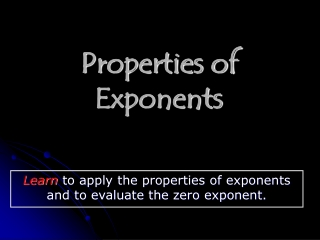Download PresentationProperties of Exponents

# Properties of Exponents - PowerPoint PPT PresentationDownload Presentation## Properties of Exponents

- - - - - - - - - - - - - - - - - - - - - - - - - - - E N D - - - - - - - - - - - - - - - - - - - - - - - - - - -
##### Presentation Transcript

1. Properties of Exponents Learn to apply the properties of exponents and to evaluate the zero exponent.

2. The factors of a power, such as 74, can be grouped in different ways. Notice the relationship of the exponents in each product. 7 • 7 • 7 • 7 = 74 (7 • 7 • 7) • 7 = 73 • 71 = 74 (7 • 7) • (7 • 7) = 72 • 72 = 74

3. MULTIPLYING POWERS WITH THE SAME BASE Numbers Algebra Words To multiply powers with the same base, keep the base and add the exponents. bm • bn = bm+ n 35• 38 = 35 + 8 = 313

4. A. 66 •63 6 6 + 3 6 9 n 5 + 7 n 12 Multiplying Powers with the Same Base Multiply. Write the product as one power. Add exponents. B. n5 •n7 Add exponents.

5. 1 Think: 2 = 2 2 5 + 1 2 6 24 4 + 4 24 8 Multiplying Powers with the Same Base Continued Multiply. Write the product as one power. C. 25• 2 Add exponents. D.244• 244 Add exponents.

6. 5555 5 5555 5 5 5 = = = 5 •5 = 52 555 555 53 DIVIDING POWERS WITH THE SAME BASE Words Numbers Algebra To divide powers with the samebase, keep the base and subtract the exponents. 6 b 9 m = = = 6 b 6 9 – 4 m – n 5 6 b 4 n Notice what occurs when you divide powers with the same base.

7. 5 – 3 10 – 9 x 7 9 10 3 2 5 7 x x 7 7 Think: x = x 1 Dividing Powers with the Same Base Divide. Write the quotient as one power. A. Subtract exponents. B. Subtract exponents. x

8. 9 – 2 9 10 – 5 e 5 e Try This: Divide. Write the quotient as one power. 9 9 A. 9 2 Subtract exponents. 97 e 10 B. e 5 Subtract exponents.

9. 4 2 = 42 – 2 = 40 = 1 1 = 2 4 1 1 (4 • 4) (4 • 4) 4 2 = 1 = = = (4 • 4) (4 • 4) 4 2 When the numerator and denominator have the same base and exponent, subtracting the exponents results in a 0 exponent. This result can be confirmed by writing out the factors.

10. Helpful Hint 0 does not exist because 00 represents a quotient of the form But the denominator of this quotient is 0, which is impossible, since you cannot divide by 0! It is undefined! . 0n 0n 0

11. THE ZERO POWER Algebra Words Numbers The zero power of any number except 0 equals 1. 1000 = 1 a = 1, if a 0 (–7)0 = 1

12. 109 105 t9 t7 Practice Write the product or quotient as one power 1. n3n42. 3.4. 33 • 32 • 355. 8 • 88 = • n7 • 104 • t2 • 310 • 89